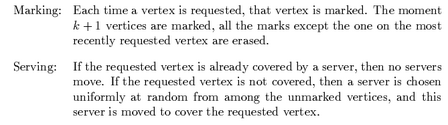## neal young / Fiat91Competitive

• Journal of Algorithms 12(4):685-699(1991)The paging problem is that of deciding which pages to keep in a memory of $$k$$ pages in order to minimize the number of page faults. This paper introduced the marking algorithm , a randomized on-line algorithm for the paging problem, and a proof that its performance guarantee is $$O(\log k)$$. This was one of the first results in the area showing that a randomized algorithm can have a strictly better performance guarantee than any deterministic on-line algorithm (no deterministic on-line algorithm can have a performance guarantee better than k).